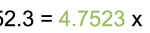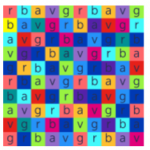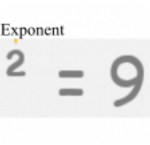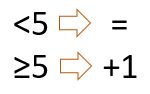Smartick is an advanced online program that teaches kids math and coding in only 15 min. a day

# I want to learn about: Number & Operations In Base Ten

Content about numbers for elementary mathematics. Exercises, tutorials, problems, and teaching resources for elementary aged children. Here you will find posts about numbers to make learning mathematics easier and more fun.

Aug14

## What Is Scientific Notation and Why Do We Use It?What is scientific notation? Why do we use it? How do we use it? How can we write a number in scientific notation? These are some questions that we’ll answer in today’s post.   What is scientific notation and why do we use it? Working with very large or small quantities usually ends up being […]

Apr05

## Learn and Practice How to Use Exponents in a Word ProblemAre you familiar with exponents (powers)? Do you know for what and how they are used? Today, we are going to see an example that will help you to solve problems with exponents or powers. If you need to review what powers are before learning how to use them in a word problem, you can […]

Mar16

## Learn How to Find Exact Square Roots and Visual ExamplesThanks for choosing Smartick to continue learning math are you ready to begin with square roots? Well, let’s go! In this week’s post, we will learn to calculate exact square roots and some visual examples where they apply. As you know, graphical visualization is always a great help in understanding and assimilating new concepts. I […]

Mar15

## Powers: What They Are and What They Are ForHave you ever heard of powers? Do you know what they are and what they’re for? In this week’s post, we are going to discover what a power is and why we use them. Powers are an abbreviated way of writing a number that’s formed by multiplying a number by itself various times. They are very useful […]

Mar03

## The Square of a Number and Exponents

In today’s post, we are going to see what the square of a number is. For example, let’s calculate the square of 3: We’re going to look at the square of any number, which we’ll call “n.” The square of the number “n” is written n2 and it’s calculated by multiplying the number by itself: […]

Feb17

## Combining Decimals and Fractions

Imagine that you have to solve the following addition problem that combines decimals and fractions: It’s not so easy to add a fraction to a decimal, right? It’s much easier to add fractions to fractions, or decimals to decimals. So, we can do this in two ways: Change the decimal to a fraction. Change the […]

Jan27

## Learn How to Do Division Word Problems with DecimalsToday we are going to review division word problems with decimals. Does it seem difficult? Well, it’s not! Solving division word problems with decimals is the same as solving them with whole numbers. I’m going to show you! You can review what a decimal number is here. Every time we solve a word problem, the first […]

Jan19

## Learn about the Importance of the Parentheses in PowersIn this entry, we will learn the importance of the parentheses when performing calculations with signs (negative) in the powers. We all know that multiplication is an abbreviated form of writing a reiterated sum. For example, 2 + 2 + 2 + 2 + 2 can be more simply written as 2 x 5. In […]